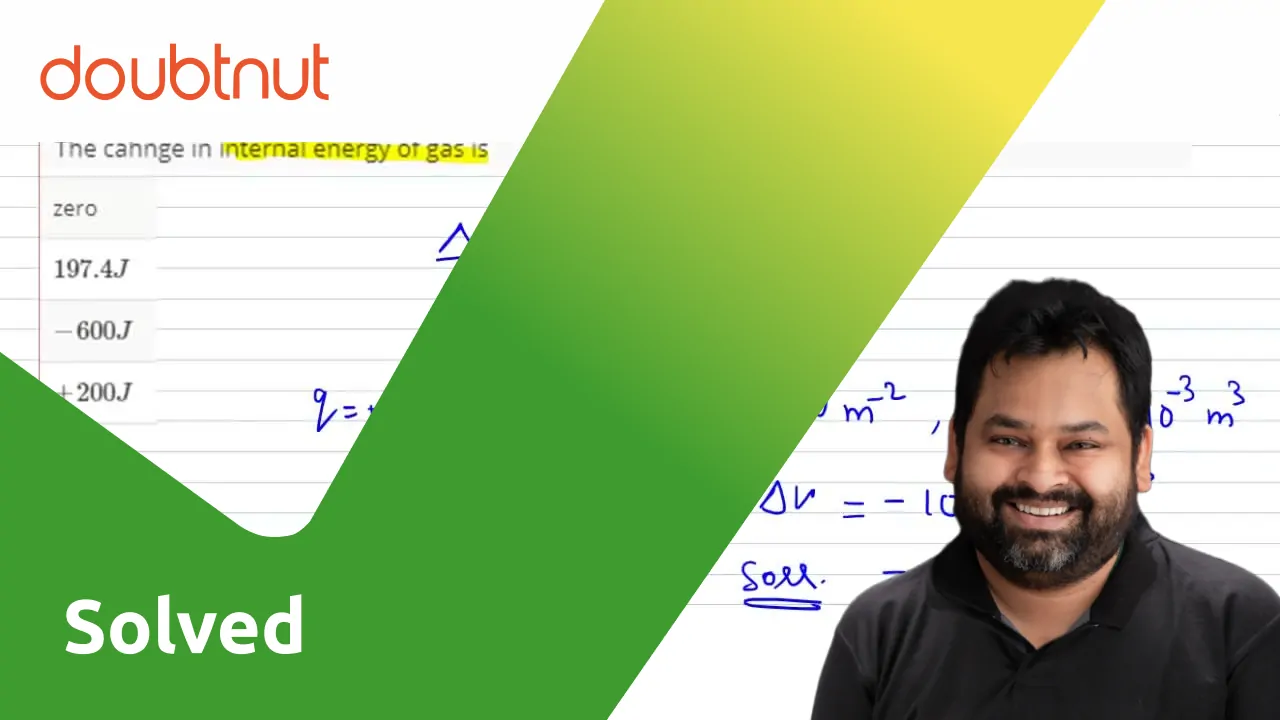Doubtnut App - Get 50 Lakh+ video solutions better than GoogleInstall Now

# A gas absorbs 400J of heat and expands by 2×10−3m3 against a constant pressure of 105Nm−2. The cahnge in internal energy of gas is

A

zero

B

197.4J

C

600J

D

+200J

Text Solution
Verified by Experts

## ΔE=q+W,q=+400J W=−PΔV=105Nm−2×10−3m3 =200Nm as 1J=Nm∴ΔE=+400−200=200J